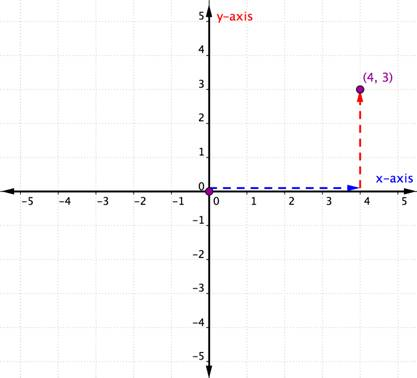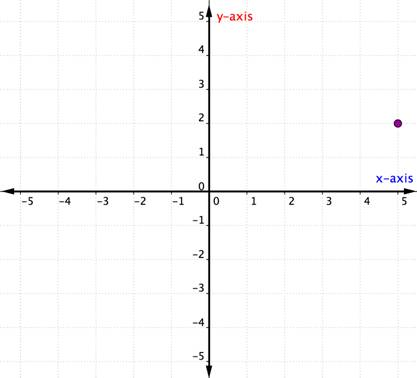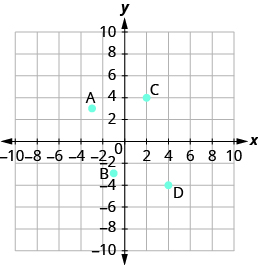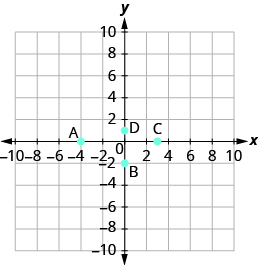## Identifying Points on a Rectangular Coordinate System

### Learning Outcomes

• Identify points on a graph

In algebra, being able to identify the coordinates of a point shown on a graph is just as important as being able to plot points. To identify the x-coordinate of a point on a graph, read the number on the x-axis directly above or below the point. To identify the y-coordinate of a point, read the number on the y-axis directly to the left or right of the point. Remember, to write the ordered pair using the correct order $\left(x,y\right)$.

Consider the point below.To identify the location of this point, start at the origin $(0, 0)$ and move right along the x-axis until you are under the point. Look at the label on the x-axis. The $4$ indicates that, from the origin, you have traveled four units to the right along the x-axis. This is the x-coordinate, the first number in the ordered pair.

From $4$ on the x-axis move up to the point and notice the number with which it aligns on the y-axis. The $3$ indicates that, after leaving the x-axis, you traveled $3$ units up in the vertical direction, the direction of the y-axis. This number is the y-coordinate, the second number in the ordered pair. With an x-coordinate of $4$ and a y-coordinate of $3$, you have the ordered pair $(4, 3)$.

Let’s look at another example.

### Example

Describe the point shown as an ordered pair.## Describe the point shown as an ordered pair

### example

Name the ordered pair of each point shown:Solution
Point A is above $-3$ on the $x\text{-axis}$, so the $x\text{-coordinate}$ of the point is $-3$. The point is to the left of $3$ on the $y\text{-axis}$, so the $y\text{-coordinate}$ of the point is $3$. The coordinates of the point are $\left(-3,3\right)$.

Point B is below $-1$ on the $x\text{-axis}$, so the $x\text{-coordinate}$ of the point is $-1$. The point is to the left of $-3$ on the $y\text{-axis}$, so the $y\text{-coordinate}$ of the point is $-3$. The coordinates of the point are $\left(-1,-3\right)$.

Point C is above $2$ on the $x\text{-axis}$, so the $x\text{-coordinate}$ of the point is $2$. The point is to the right of $4$ on the $y\text{-axis}$, so the $y\text{-coordinate}$ of the point is $4$. The coordinates of the point are $\left(2,4\right)$.

Point D is below $4$ on the $x-\text{axis}$, so the $x\text{-coordinate}$ of the point is $4$. The point is to the right of $-4$ on the $y\text{-axis}$, so the $y\text{-coordinate}$ of the point is $-4$. The coordinates of the point are $\left(4,-4\right)$.

### example

Name the ordered pair of each point shown:### try it

Watch the following video for another example of how to determine the ordered pair for points on the coordinate plane.

## Contribute!

Did you have an idea for improving this content? We’d love your input.